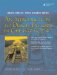# Valid Pointer Operations

Here is a list of the operations that can properly be performed with pointers.

Creation The initial value of a pointer has three possible sources:

• A const pointer such as an array name
• A value obtained by a dynamic memory allocation operator (e.g., new)

Assignment

• A pointer can be assigned the address stored by a pointer of the same type or of a derived type.
• A variable of type void* can be assigned a pointer of any type without an explicit cast.
• A (non-void*) pointer can be assigned the address stored by a pointer of a different (and non-derived) type only with an explicit cast.
• An array name is a const pointer and cannot be assigned to.
• A NULL pointer (value 0) can always be assigned to any pointer. (Note: Stroustrup recommends that 0 be used instead of NULL.)

Arithmetic

• Incrementing and decrementing a pointer: p++ and p--
• Adding or subtracting an integer: p + k and p - k
• Such expressions are defined only if the resulting pointer value is within the range of the same array. The only exception to this rule is that a pointer is allowed to point to the memory cell that is one position beyond the end of the array as long as no attempt is made to dereference that address.
• For subtraction, two pointers that point to two members of an array can be subtracted, yielding an int that represents the number of array elements between the two members.

Comparison

• Pointers to entries of the same array can be compared with ==, !=, <, >, etc.
• Any pointer can be compared with 0.

Indirection

• If p is a pointer of type T*, then *p is a variable of type T and can be used on the left side of an assignment.

Indexing

• A pointer p can be used with an array index operator p[i] where i is an int.
• The compiler interprets such an expression as *(p+i).
• Indexing makes sense and is defined only in the context of an array, but the compiler will not prevent its use with non-array pointers where the results are undefined.

The following bit of code in Example 22.6 demonstrates this last point rather clearly.

Example 22.6. src/arrays/pointerIndex.cpp

 ```#include using namespace std; int main() { int x = 23; int* px = &x; cout << "px = " << px << endl; cout << "px = " << px << endl; cout << "px[-1] = " << px[-1] << endl; return 0; } Output: src/arays> g++ pointerIndex.cc // compile & run on a Sun station src/arays> a.out px = 23 px = 5 px[-1] = -268437516 src/arays> g++ pointerIndex.cc // compile & run on a Linux box src/arays> ./a.out px = 23 px = -1073743784 px[-1] = -1073743852 src/arays> ```An Introduction to Design Patterns in C++ with Qt 4
ISBN: 0131879057
EAN: 2147483647
Year: 2004
Pages: 268